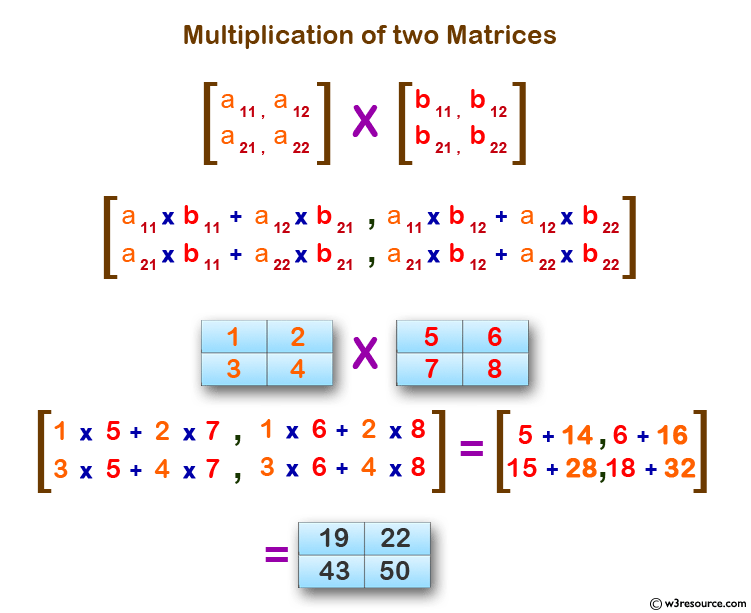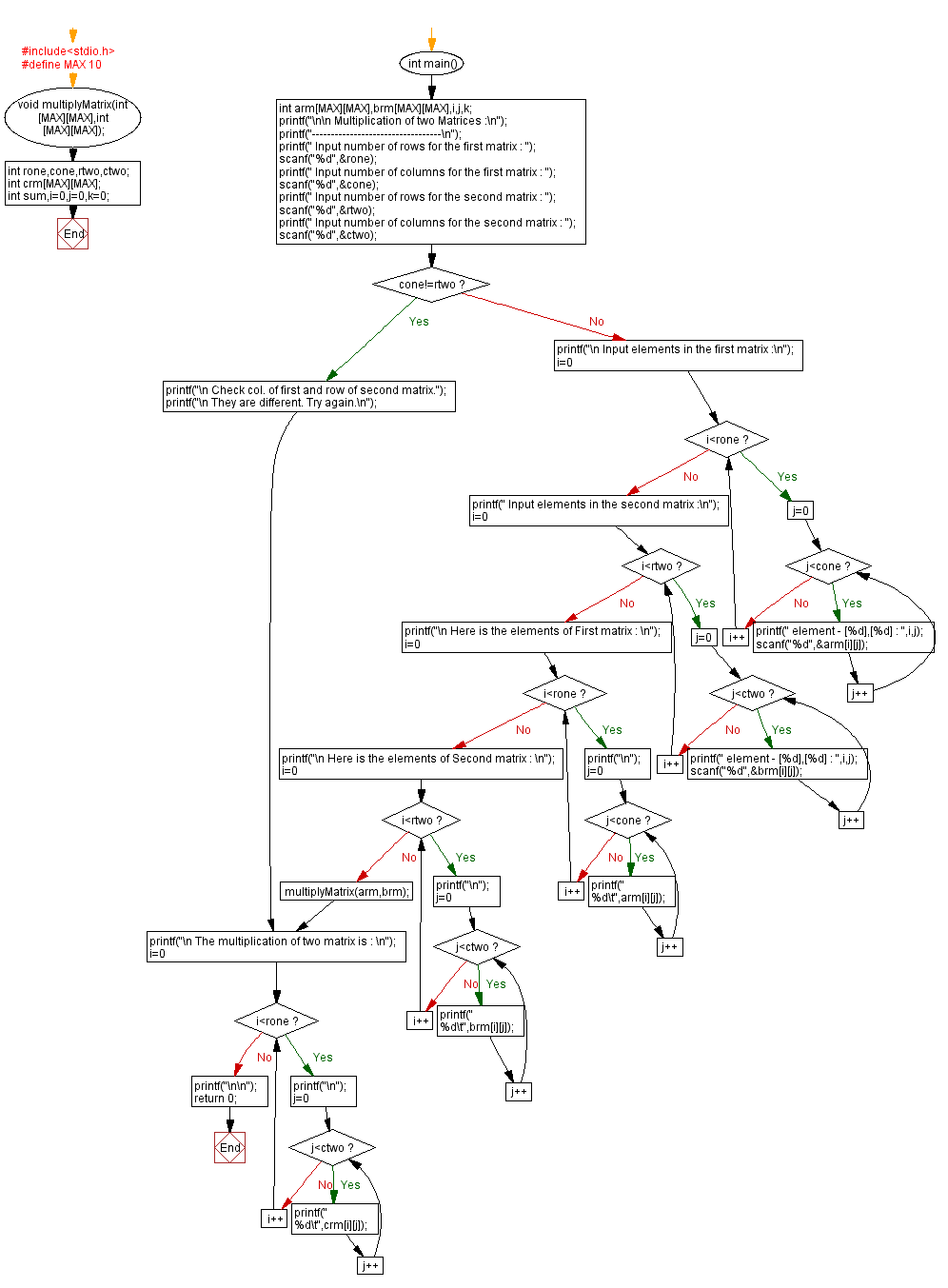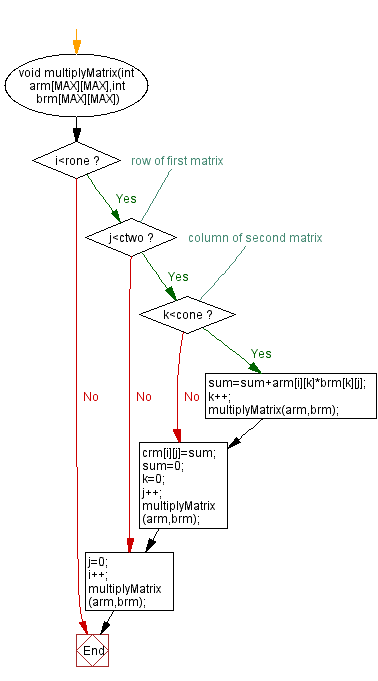﻿ C : Multiplication of two Matrices using recursion

# C Exercises: Multiplication of two Matrices

## C Recursion : Exercise-15 with Solution

Write a C program to multiply two matrices using recursion.

Pictorial Presentation:Sample Solution:

C Code:

``````#include<stdio.h>
#define MAX 10
void multiplyMatrix(int [MAX][MAX],int [MAX][MAX]);
int rone,cone,rtwo,ctwo;
int crm[MAX][MAX];
int sum,i=0,j=0,k=0;
int main()
{
int arm[MAX][MAX],brm[MAX][MAX],i,j,k;

printf("\n\n Multiplication of two Matrices :\n");
printf("----------------------------------\n");
printf(" Input number of rows for the first matrix : ");
scanf("%d",&rone);
printf(" Input number of columns for the first matrix : ");
scanf("%d",&cone);

printf(" Input number of rows for the second matrix : ");
scanf("%d",&rtwo);
printf(" Input number of columns for the second matrix : ");
scanf("%d",&ctwo);
if(cone!=rtwo)
{
printf("\n Check col. of first and row of second matrix.");
printf("\n They are different. Try again.\n");
}
else
{
printf("\n Input elements in the first matrix :\n");
for(i=0;i<rone;i++){
for(j=0;j<cone;j++){
printf(" element - [%d],[%d] : ",i,j);
scanf("%d",&arm[i][j]);}}
printf(" Input elements in the second matrix :\n");
for(i=0;i<rtwo;i++){
for(j=0;j<ctwo;j++){
printf(" element - [%d],[%d] : ",i,j);
scanf("%d",&brm[i][j]);}}
printf("\n Here is the elements of First matrix : \n");
for(i=0;i<rone;i++)
{
printf("\n");
for(j=0;j<cone;j++)
{
printf(" %d\t",arm[i][j]);
}
}
printf("\n Here is the elements of Second matrix : \n");
for(i=0;i<rtwo;i++)
{
printf("\n");
for(j=0;j<ctwo;j++)
{
printf(" %d\t",brm[i][j]);
}
}
multiplyMatrix(arm,brm);
}
printf("\n The multiplication of two matrix is : \n");
for(i=0;i<rone;i++)
{
printf("\n");
for(j=0;j<ctwo;j++)
{
printf(" %d\t",crm[i][j]);
}
}
printf("\n\n");
return 0;
}
void multiplyMatrix(int arm[MAX][MAX],int brm[MAX][MAX])
{
if(i<rone)
{ //row of first matrix
if(j<ctwo)
{  //column of second matrix
if(k<cone)
{
sum=sum+arm[i][k]*brm[k][j];
k++;
multiplyMatrix(arm,brm);
}
crm[i][j]=sum;
sum=0;
k=0;
j++;
multiplyMatrix(arm,brm);
}
j=0;
i++;
multiplyMatrix(arm,brm);
}
}
```
```

Sample Output:

``` Multiplication of two Matrices :
----------------------------------
Input number of rows for the first matrix : 2
Input number of columns for the first matrix : 1
Input number of rows for the second matrix : 1
Input number of columns for the second matrix : 2
Input elements in the first matrix :
element - , : 1
element - , : 2
Input elements in the second matrix :
element - , : 3
element - , : 4
Here is the elements of First matrix :
1
2
Here is the elements of Second matrix :
3       4
The multiplication of two matrix is :
3       4
6       8
```
``` Multiplication of two Matrices :
----------------------------------
Input number of rows for the first matrix : 2
Input number of columns for the first matrix : 2
Input number of rows for the second matrix : 2
Input number of columns for the second matrix : 2
Input elements in the first matrix :
element - , : 1
element - , : 2
element - , : 3
element - , : 4
Input elements in the second matrix :
element - , : 5
element - , : 6
element - , : 7
element - , : 8
Here is the elements of First matrix :
1       2
3       4
Here is the elements of Second matrix :
5       6
7       8
The multiplication of two matrix is :
19      22
43      50
```

Explanation:

```void multiplyMatrix(int arm[MAX][MAX],int brm[MAX][MAX])
{
if(i<rone)
{ //row of first matrix
if(j<ctwo)
{  //column of second matrix
if(k<cone)
{
sum=sum+arm[i][k]*brm[k][j];
k++;
multiplyMatrix(arm,brm);
}
crm[i][j]=sum;
sum=0;
k=0;
j++;
multiplyMatrix(arm,brm);
}
j=0;
i++;
multiplyMatrix(arm,brm);
}
}
```

The above function ‘multiplyMatrix ()’ uses three static variables i, j, and k to keep track of the current row and column being processed in each matrix. The variable sum is used to accumulate the product of each element in the row of the first matrix and the corresponding element in the column of the second matrix.

The function checks if the row index i is less than the number of rows rone in the first matrix.

• If it is, it checks if the column index j is less than the number of columns ctwo in the second matrix.
• If it is, it checks if the variable k is less than the number of columns cone in the first matrix.
• If it is, the function multiplies the corresponding elements of the two matrices, adds the result to sum, and increments k.
• It then calls itself recursively to continue processing the current row and column.
• If k is equal to cone, the function stores the result in the third matrix crm, resets sum and k, increments j, and calls itself recursively to continue processing the current row and column.
• If j is equal to ctwo, the function resets j, increments i, and calls itself recursively to continue processing the current row and column.

The function continues to call itself recursively until all the elements of the matrices have been processed.

Time complexity and space complexity:

The time complexity of the function is O(n^3) where n is the number of rows or columns in the matrix. This is because the function involves three nested loops to iterate through the rows and columns of the two matrices.

The space complexity of the function is O(n^2) as it requires two-dimensional arrays to store the input matrices and the resulting product matrix.

Flowchart:C Programming Code Editor:

Have another way to solve this solution? Contribute your code (and comments) through Disqus.

What is the difficulty level of this exercise?

Test your Programming skills with w3resource's quiz.

﻿

## C Programming: Tips of the Day

__FILE__ macro shows full path

```#include <string.h>

#define __FILENAME__ (strrchr(__FILE__, '/') ? strrchr(__FILE__, '/') + 1 : __FILE__)
For Windows use '\\' instead of '/'.
```

Ref : https://bit.ly/3iEWRoT

We are closing our Disqus commenting system for some maintenanace issues. You may write to us at reach[at]yahoo[dot]com or visit us at Facebook